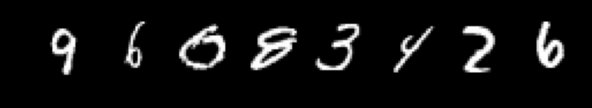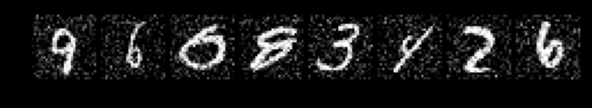# How to add noise to image in denoising autoencoders

I want to implement denoising autoencoder in pytorch.
when I am in the training loop:

``````for inputs,labels in trainloader:
outputs = net(noised_inputs)
loss = loss_fn(outputs,inputs)
loss.backward()
opt.step()
``````

Please tell me how to implement this add_noise function which can add gaussian noise to the entire batch input of images

You can use the `torch.randn_like()` function to create a noisy tensor of the same size of input. Then add it.

``````def add_noise(inputs):
noise = torch.randn_like(inputs)
return inputs + noise
``````

It worked!!! also we can multiply it with factor like 0.2 to reduce the noise

``````def add_noise(inputs):
noise = torch.randn_like(inputs)*0.2
return inputs + noise
``````

Original Image:Noised Image: